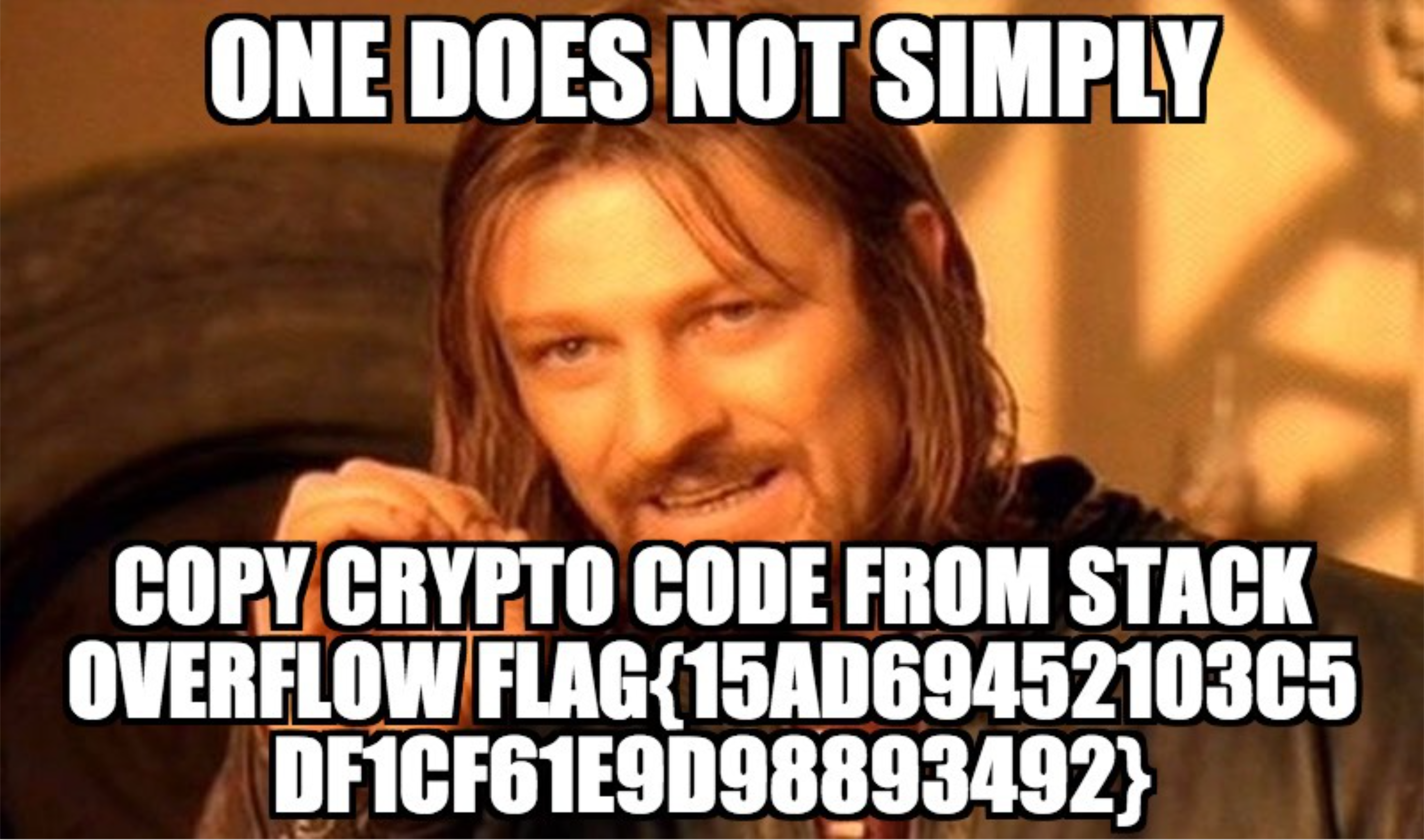## SHA2017 - Stack Overflow (Crypto 100)

This challenge was accompanied by two files, an encrypted pdf file named flag.pdf.enc and the following python file named encrypt.py.

``````import os, sys
from Crypto.Cipher import AES

fn = sys.argv

# Secure CTR mode encryption using random key and random IV, taken from
# http://stackoverflow.com/questions/3154998/pycrypto-problem-using-aesctr
secret = os.urandom(16)
crypto = AES.new(os.urandom(32), AES.MODE_CTR, counter=lambda: secret)

encrypted = crypto.encrypt(data)
open(fn+'.enc','wb').write(encrypted)
``````

The stack overflow post that this is taken from hints at the issue, but a clearer picture comes from the PyCrypto documentation for AES. The important part is the counter parameter. The code is attempting to use CTR mode. The way that CTR mode is supposed to work, as it says in the Wikipedia article, is that the keystream is generated by encrypting successive values of a counter. That is, the AES encryption function is only used to encrypt the counter values, and the output is XOR’d with the plaintext in order to encrypt it.

The counter parameter in PyCrypto AES is supposd to be a function that returns the successive values of the counter each time it is called. That is, the first time it is called, it should return the initial counter value. The second time, it should return counter + 1. And so on. However, in this code, it declares the counter function as a lambda function that returns the static value `secret`.

Since the same counter value is used for each block, then each block is XOR’d with the same pseudo-random block. What this means is that if we know an entire block, we can XOR it with the same ciphertext block and get the key block out. We can then use this to XOR with each block of the ciphertext to get the complete plaintext out. So then the question is, what block do we know?

Fortunately, there is a lot in a pdf file that is predictable or known. By looking at the PDF file format we see that the beginning starts with `%PDF-1.` followed by the version number, which we don’t know, and a newline character and another `%`. The end of the file should be `startxref\n` followed by a 6 or 7 digit number (in ASCII) followed by `\n%%EOF`. So we know bits and pieces but not a full block. However, what we can do is use the bits we know to get parts of the key and use that to decrypt the same parts of the rest of the block, and repeat, building up our knowledge of a full block.

I used a small script to accomplish this that I will include below, but the blocks that I figured out were added to previous blocks, so the code is the final stage of a multistep process. The main part that helped me towards the end was the BitsPerComponent. That was a keyword that appeared in a bunch of other pdfs that I examined in order to figure out the structure and content. The beginning and end of the keyword was decrypted, and it was clear what the word was, so I was able to filling the rest and get a full block. This is the code that I used.

``````#!/usr/bin/python
import sys

#copied from here :https://stackoverflow.com/questions/2612720/how-to-do-bitwise-exclusive-or-of-two-strings-in-python
def sxor(s1,s2):
# convert strings to a list of character pair tuples
# go through each tuple, converting them to ASCII code (ord)
# perform exclusive or on the ASCII code
# then convert the result back to ASCII (chr)
# merge the resulting array of characters as a string
return ''.join(chr(ord(a) ^ ord(b)) for a,b in zip(s1,s2))

parts = [data[i:i+16] for i in range(0, len(data), 16)]

firstblock="%PDF-1.4\x0a%\x00\x00\x03 ob"
fourthblock="\x6f\x07\x20\x0a\x3e\x3e\x0aendobj\x3b\x12\x13"
thirtysixthblock=" R\x0a/BitsPerCompo"
lastblock="xref\x0a1469999\x0a%%E"
#print len(parts) 6864
#print parts
key = sxor(firstblock, parts)
#key = sxor(lastblock, parts)
#key = sxor(fourthblock, parts)
key = sxor(thirtysixthblock, parts)
#sys.stdout.write(sxor(key, parts))
for part in parts:
line = sxor(key, part)
#print len(line)
sys.stdout.write(line)
``````

A few notes. I had started out using the `print` statement to output the decrypted file, but python automatically adds a newline. You can suppress this with a comma at the end of the print statement, but it still outputs a space at the end, so the blocks weren’t lining up properly. This drove me crazy for a while, until I switched to `sys.stdout.write()`.

Once I got a whole block, I was able to decrypt the entire file. The resulting pdf was the following image.By the way, that is one of my pet peeves in CTFs. When the flag is long and random, and included in an image, so you can’t cut and paste it. :)